# Homologously Collapsing Polytropic Spheres

## Review of Goldreich and Weber (1980)

In an accompanying discussion, we have reviewed the self-similar, dynamical model that Peter Goldreich & Stephen Weber (1980, ApJ, 238, 991) developed to describe the near-homologous collapse of stellar cores that obey an equation of state having an adiabatic index,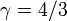$~\gamma=4/3$ — and polytropic index,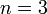$~n=3$. Here we examine whether or not this self-similar collapse model can be extended to configurations with arbitrary polytropic index.

## Governing Equations

We begin with the set of principal governing equations that are already written in terms of a stream function and a time-varying radial coordinate,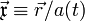$~\vec\mathfrak{x} \equiv \vec{r}/a(t)$, as developed in the accompanying discussion of Goldreich & Weber's (1980) work. The continuity equation, the Euler equation, and the Poisson equation are, respectively,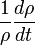$~\frac{1}{\rho} \frac{d\rho}{dt}$$~=$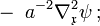$~-~ a^{-2} \nabla_\mathfrak{x}^2 \psi \, ;$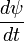$~\frac{d\psi}{dt}$$~=$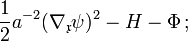$~\frac{1}{2} a^{-2} ( \nabla_\mathfrak{x} \psi )^2 - H - \Phi \, ;$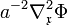$~a^{-2}\nabla_\mathfrak{x}^2 \Phi$$~=$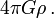$~4\pi G \rho \, .$

Following Goldreich & Weber (1980), the normalization length scale,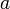$~a$, that appears in this set of equations is the same length scale that is used in deriving the Lane-Emden equation, namely,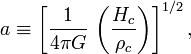$a \equiv \biggl[\frac{1}{4\pi G}~ \biggl( \frac{H_c}{\rho_c} \biggr)\biggr]^{1/2} \, ,$

where the subscript, "c", denotes central values and, as presented in our introductory discussion of barotropic supplemental relations,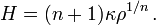$~H = (n+1) \kappa \rho^{1/n} \, .$

But, unlike Goldreich & Weber, we will leave the polytropic index unspecified. Inserting this equation of state expression into the definition of the normalization length scale leads to,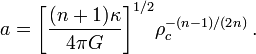$a = \biggl[\frac{(n+1)\kappa}{4\pi G}\biggr]^{1/2} \rho_c^{-(n-1)/(2n)} \, .$

Following Goldreich & Weber, we allow the normalizing scale length to vary with time and adopt an accelerating coordinate system with a time-dependent dimensionless radial coordinate,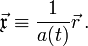$~\vec\mathfrak{x} \equiv \frac{1}{a(t)} \vec{r} \, .$

(The spatial operators in the above set of principal governing equations exhibit a subscript,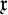$~\mathfrak{x}$, indicating the adoption of this accelerating coordinate system.) This, in turn, reflects a time-varying central density; specifically,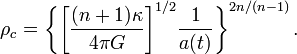$\rho_c = \biggl\{ \biggl[\frac{(n+1)\kappa}{4\pi G}\biggr]^{1/2} \frac{1}{a(t)} \biggr\}^{2n/(n-1)} \, .$

Next, we normalize the density by the central density, defining a dimensionless function,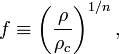$f \equiv \biggl( \frac{\rho}{\rho_c} \biggr)^{1/n} \, ,$

which is in line with the formulation and evaluation of the Lane-Emden equation, where the primary dependent structural variable is the dimensionless polytropic enthalpy,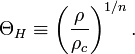$\Theta_H \equiv \biggl( \frac{\rho}{\rho_c} \biggr)^{1/n} \, .$

And we normalize both the gravitational potential and the enthalpy to the square of the central sound speed,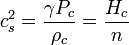$~c_s^2 = \frac{\gamma P_c}{\rho_c} = \frac{H_c}{n}$$~=$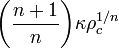$~\biggl( \frac{n+1}{n} \biggr) \kappa \rho_c^{1/n}$$~=$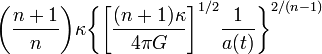$~\biggl( \frac{n+1}{n} \biggr) \kappa \biggl\{ \biggl[\frac{(n+1)\kappa}{4\pi G}\biggr]^{1/2} \frac{1}{a(t)} \biggr\}^{2/(n-1)}$$~=$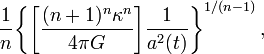$~\frac{1}{n} \biggl\{ \biggl[\frac{(n+1)^n \kappa^n}{4\pi G}\biggr] \frac{1}{a^2(t)} \biggr\}^{1/(n-1)} \, ,$

giving,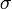$~\sigma$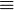$~\equiv$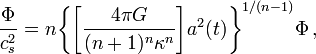$~\frac{\Phi}{c_s^2} = n \biggl\{ \biggl[\frac{4\pi G}{(n+1)^n \kappa^n}\biggr] a^2(t) \biggr\}^{1/(n-1)} \Phi \, ,$

and,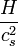$~\frac{H}{c_s^2}$$~=$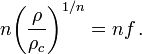$~n \biggl( \frac{\rho}{\rho_c} \biggr)^{1/n} = nf \, .$

With these additional scalings, the continuity equation becomes,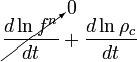$~\cancelto{0}{\frac{d\ln f^n}{dt}} + \frac{d\ln \rho_c}{dt}$$~=$$~-~ a^{-2} \nabla_\mathfrak{x}^2 \psi \, ;$

the Euler equation becomes,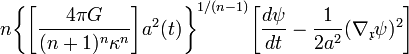$~ n \biggl\{ \biggl[\frac{4\pi G}{(n+1)^n \kappa^n}\biggr] a^2(t) \biggr\}^{1/(n-1)} \biggl[ \frac{d\psi}{dt} - \frac{1}{2a^2} ( \nabla_\mathfrak{x} \psi )^2 \biggr]$$~=$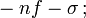$~ - n f - \sigma \, ;$

and the Poisson equation becomes,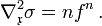$\nabla_\mathfrak{x}^2 \sigma = n f^n \, .$

## Homologous Solution

Goldreich & Weber (1980) discovered that the governing equations admit to an homologous, self-similar solution if they adopted a stream function of the form,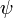$~\psi$$~=$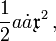$~\frac{1}{2}a \dot{a} \mathfrak{x}^2 \, ,$

which, when acted upon by the various relevant operators, gives,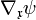$~\nabla_\mathfrak{x}\psi$$~=$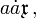$~a \dot{a} \mathfrak{x} \, ,$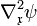$~\nabla^2_\mathfrak{x}\psi$$~=$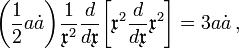$~ \biggl( \frac{1}{2}a \dot{a} \biggr) \frac{1}{\mathfrak{x}^2} \frac{d}{d\mathfrak{x}} \biggl[\mathfrak{x}^2 \frac{d}{d\mathfrak{x}} \mathfrak{x}^2 \biggr] = 3 a \dot{a} \, ,$$~\frac{d\psi}{dt}$$~=$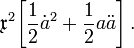$~\mathfrak{x}^2 \biggl[ \frac{1}{2}\dot{a}^2 + \frac{1}{2}a\ddot{a} \biggr] \, .$

This stream-function appears to be a reasonable choice, as well, in the more general case that we are considering, in which case the radial velocity profile is, as in the Goldreich & Weber derivation,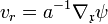$~v_r = a^{-1}\nabla_\mathfrak{x} \psi$$~=$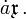$~\dot{a}\mathfrak{x} \, .$

Also, in our more general case, the continuity equation gives,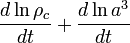$~\frac{d\ln \rho_c}{dt} + \frac{d\ln a^3}{dt}$$~=$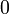$~0$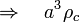$\Rightarrow~~~ a^3\rho_c$$~=$ constant,

independent of time. But the Euler equation becomes,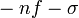$~ - n f - \sigma$$~=$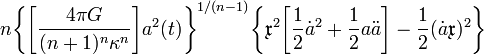$~ n \biggl\{ \biggl[\frac{4\pi G}{(n+1)^n \kappa^n}\biggr] a^2(t) \biggr\}^{1/(n-1)} \biggl\{ \mathfrak{x}^2 \biggl[ \frac{1}{2}\dot{a}^2 + \frac{1}{2}a\ddot{a} \biggr] - \frac{1}{2} ( \dot{a} \mathfrak{x} )^2 \biggr\}$$~=$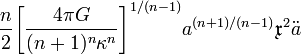$~ \frac{n}{2} \biggl[\frac{4\pi G}{(n+1)^n \kappa^n}\biggr]^{1/(n-1)} a^{(n+1)/(n-1)} \mathfrak{x}^2 \ddot{a}$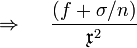$~\Rightarrow~~~~ \frac{(f + \sigma/n)}{\mathfrak{x}^2}$$~=$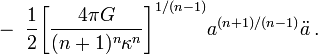$~ -~\frac{1}{2} \biggl[\frac{4\pi G}{(n+1)^n \kappa^n}\biggr]^{1/(n-1)} a^{(n+1)/(n-1)} \ddot{a} \, .$

Because everything on the lefthand side of this scaled Euler equation depends only on the dimensionless spatial coordinate,$~\mathfrak{x}$, while everything on the righthand side depends only on time — via the parameter,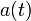$~a(t)$ — both expressions must equal the same (dimensionless) constant. We will follow the lead of Goldreich & Weber (1980) and call this constant,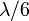$~\lambda/6$. From the terms on the lefthand side, we conclude that the dimensionless gravitational potential is,$~\sigma$$~=$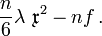$~\frac{n}{6} \lambda ~\mathfrak{x}^2 - nf \, .$

Inserting this expression into the Poisson equation gives,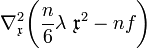$~\nabla^2_\mathfrak{x} \biggl( \frac{n}{6} \lambda ~\mathfrak{x}^2 - nf \biggr)$$~=$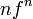$~nf^n$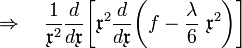$~\Rightarrow~~~\frac{1}{\mathfrak{x}^2} \frac{d}{d\mathfrak{x}} \biggl[ \mathfrak{x}^2 \frac{d}{d\mathfrak{x}} \biggl(f - \frac{\lambda}{6} ~\mathfrak{x}^2 \biggr)\biggr]$$~=$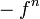$~-f^n$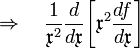$~\Rightarrow~~~\frac{1}{\mathfrak{x}^2} \frac{d}{d\mathfrak{x}} \biggl[ \mathfrak{x}^2 \frac{df}{d\mathfrak{x}} \biggr]$$~=$$~\lambda - f^n \, ,$

which becomes the familiar Lane-Emden equation for arbitrary n when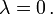$~\lambda = 0 \, .$

From the terms on the righthand side we conclude, furthermore, that the nonlinear differential equation governing the time-dependent variation of the scale length,$~a$, is,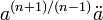$~ a^{(n+1)/(n-1)} \ddot{a}$$~=$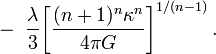$~-~\frac{\lambda}{3} \biggl[ \frac{(n+1)^n \kappa^n}{4\pi G} \biggr]^{1/(n-1)} \, .$

 © 2014 - 2020 by Joel E. Tohline |   H_Book Home   |   YouTube   | Appendices: | Equations | Variables | References | Ramblings | Images | myphys.lsu | ADS |